# Determining Future Position of Uniform Circular Motion

• I
Summary:
Determining the future position of an object undergoing uniform circular motion
Hello,

Apologies if this is in the wrong section, it's related to circles so I figured Geometry was the best place. I found a very good example online that explains how to determine a future position of an object undergoing uniform circular motion:

(Note that they made a mistake by writing 0.095j as 0.95j on the diagram).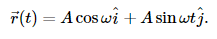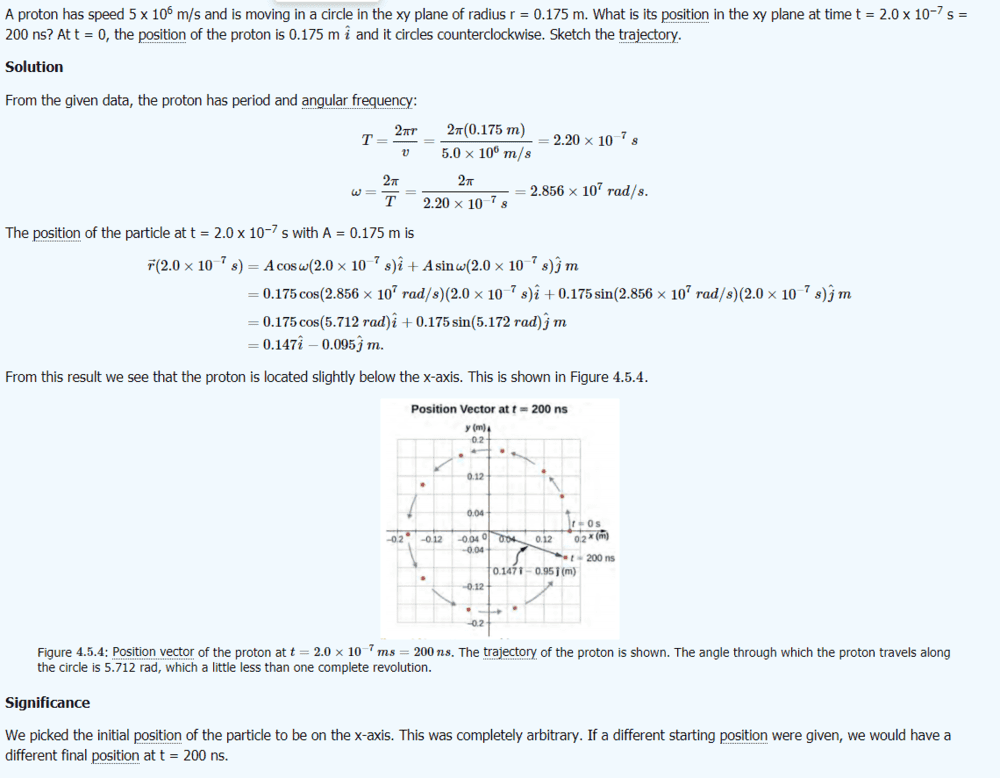However I am confused as to how a different starting position would affect the calculations above? The example has a start point of (0.175i + 0j). However, what if the particle started at 0.147i + 0.095j (first pink dot above the positive x-axis)? Can anyone please explain how this would alter the calculation? Clearly A, omega and t are the same, so how is the calculation different?

Last edited:

## Answers and Replies

The formula ## r = A \cos(\omega t)\hat i + A \sin(\omega t) \hat j ## now gives ## r(0) = 0.175 \hat i + 0 \hat j##, the formula needs to be manipulated such that you get ## r(0) = 0.147 \hat i + 0.095 \hat j##.

The formula ## r = A \cos(\omega t)\hat i + A \sin(\omega t) \hat j ## now gives ## r(0) = 0.175 \hat i + 0 \hat j##, the formula needs to be manipulated such that you get ## r(0) = 0.147 \hat i + 0.095 \hat j##.
I'm afraid I don't understand what you mean. I don't see how the equation factors in the start position. In my own use case, I know the start position, A, omega and t, and I need to find the position after t.

The startposition is whatever the formula gives for ##t = 0##. Do you know how to shift a graph along the x axis? So if you have a function ##f = f(x)## with ##f(x_0) = 0##, do you know how to change the function to get ##f(x_1) = 0## for ##x_1 \neq x_0##?

The startposition is whatever the formula gives for ##t = 0##. Do you know how to shift a graph along the x axis? So if you have a function ##f = f(x)## with ##f(x_0) = 0##, do you know how to change the function to get ##f(x_1) = 0## for ##x_1 \neq x_0##?
Ahh okay I see what you mean. But no I'm afraid I do not know how to change the function, can you please explain?

I need to be able to find the position after a variable time t.

The more general form of the formula is ## r(t) = A \cos⁡(\omega(t-t_0))\hat i + A \sin⁡(\omega(t-t_0))\hat j##. Now you need to find a ##t_0## for which ##r(0) = 0.147 \hat i +0.095 \hat j##

The more general form of the formula is ## r(t) = A \cos⁡(\omega(t-t_0))\hat i + A \sin⁡(\omega(t-t_0))\hat j##. Now you need to find a ##t_0## for which ##r(0) = 0.147 \hat i +0.095 \hat j##
Okay, thank you for explaining this one step at a time. Once I have the t0 that you specify, how is that used to find the new position?

If you have the correct ##t_0##, then you have the evolution of the electron in time for the starting position you specified. And you can see how it evolves over time. So after a similar time as in the original problem, this time the electron will be at a different place.

If you have the correct ##t_0##, then you have the evolution of the electron in time for the starting position you specified. And you can see how it evolves over time. So after a similar time as in the original problem, this time the electron will be at a different place.
Thank you for the help Arjan. I'm probably being very dim but I have found t0 (in the example above I get t0 as (2.0081x10^-8).

Are you saying to then plug this value into the equation you specified in #6, where t = (2x10^-7) and t0 is the new value I just found? This doesn't seem to be giving me the right answer.

What answer did you get, and why is it wrong you think?

So, just to be clear on what we are trying to do here: the problem you quoted said, correctly, that they've chosen that at the initial condition (i.e. t = 0) the electron started at the positive x-axis, which is indeed completely arbitrary. They also stated correctly that if a different starting position was given, a different final position (after 200ns) would be the result.

You've asked the question how a different starting position would affect the calculations. The answer is the equation from #6. So, using t0 you can change the initial position of the electron (the initial position is the position at t=0), and as a result the final position after 200ns will also be different.

By the way, when I use ##t_0 = 2.0081\cdot 10^{-8}## I get ##r(0) = 0.147 \hat i - 0.095 \hat j##. Which is not what you wanted. You wanted ##r(0) = 0.147 \hat i + 0.095 \hat j##.

So in that sense it is wrong :)

Thanks Arjen, yes I agree with your last post but think I am still fundamentally missing something in my understanding.

When I use t0 = -2.0081 x 10-8, my r(0) = 0.147i + 0.095j. I understand what has happened here, I set the new start position and solved for t(0).

But the whole point is for me to find a future position. Say I now want to find r(200ns) with a start position of 0.147i + 0.095j. When I use Equation #6, I set t = 200ns and t0 = 2.0081 x 10-8. I expect this to give me 0.175i + 0j but it does not.

Instead I get 0.072i - 0.159j, which is plainly wrong.

Oh no! I think I was using the t0 with the wrong polarity! When I repeat the calculation with t0 = -2.0081 x 10-8, I get r(200ns) = 0.175i + 0.0004j, which is correct!

Thank you so much for persisting with me

No problem :)

Another approach to the problem is to use a rotation operator on the system. That is, you can represent the system in rotated coordinates. To rotate the system counter clockwise the operator is:
$$\begin{pmatrix} x' \\ y' \end{pmatrix} = \begin{pmatrix} \cos(\theta) & -\sin(\theta) \\ \sin(\theta) & \cos(\theta) \end{pmatrix} \begin{pmatrix} x \\ y \end{pmatrix}$$
With ##x=\cos(\omega t)## and ##y=\sin(\omega t)## we get for the rotated system
$$x'=\cos(\omega t)\cos(\theta)-\sin(\omega t)\sin(\theta)=\cos(\omega t + \theta)$$
$$y'=\cos(\omega t)\sin(\theta)+\sin(\omega t)\cos(\theta)=\sin(\omega t + \theta)$$
$$\vec r(t)=\cos(\omega t + \theta)\hat i + \sin(\omega t + \theta)\hat j$$

Since your initial position is ##0.147\hat i + 0.95\hat j## we have ##\theta = \tan^{-1}(\frac{0.095}{0.147})## where ##\theta =\omega t_0##

Last edited:
•Arjan82
The more general form of the formula is ## r(t) = A \cos⁡(\omega(t-t_0))\hat i + A \sin⁡(\omega(t-t_0))\hat j##. Now you need to find a ##t_0## for which ##r(0) = 0.147 \hat i +0.095 \hat j##
Hi Arjan,

I've been solving more of these problems and I had another question if that's alright. Is it correct to say that the equation you specified here is only valid for the upper right quadrant of the circle?

It seems when the start point is in the upper left quadrant, the formula changes to:

## r(t) = -A \sin(\omega(t-t_0))\hat i + A \cos(\omega(t-t_0))\hat j##

So I would need to solve r(0) according to this equation instead. Is this correct?

Similarly, for the lower left quadrant the formula becomes:

## r(t) = -A \cos(\omega(t-t_0))\hat i - A \sin(\omega(t-t_0))\hat j##

And for the lower right quadrant:

## r(t) = A \sin(\omega(t-t_0))\hat i - A \cos(\omega(t-t_0))\hat j##

Can you please advise if this is correct?

Last edited:
I don't see why this would be the case. Can you explain your reasoning?

Did you check continuity? What happens at the boundary? So if the electron is on the x or y axis? I don't think the formula I gave you and the one you say is for the upper left quadrant are continuous there. So the electron would jump from one place to the next.

I don't see why this would be the case. Can you explain your reasoning?

Did you check continuity? What happens at the boundary? So if the electron is on the x or y axis? I don't think the formula I gave you and the one you say is for the upper left quadrant are continuous there. So the electron would jump from one place to the next.
I believe it must be the case because the i and j components are both positive only in the upper right quadrant.

For example, in the upper left quadrant, the i component (x-axis co-ordinate) is clearly negative and is given by ## r(t) = -A \sin(\omega(t-t_0))\hat i ##.

I discovered this by writing a program to calculate the future positions from a given start point. If I use only the first equation (## r(t) = A \cos(\omega(t-t_0))\hat i + A \sin(\omega(t-t_0))\hat j##), the future positions turn out incorrect when the start point is in any of the other three quadrants. However, by updating the equations (as listed above) based on the quadrant, the future position always comes out correct.

What happens at the boundary is an interesting question - I don't know actually know the answer. I cannot get a point to lie on the boundary since I am dealing with floating point numbers and the position is always shifted either side by a tiny order of magnitude.

Last edited:
But the sine becomes negative at ##\pi/2## right? So the ##x## component becomes negative if ##\pi /2 < \omega(t-t_0) < 3\pi / 2 ##. So the the ##x## coordinate indeed becomes negative as it should. I don't see why you should alter the equations for that. Can you show me the program?

Here is the function that I am describing:

Uniform Motion Function:
public static Vector3 UniformMotionFuturePosition(Vector3 startPosition, Vector3 orbitCentre, float orbitRadius, float orbitPeriod, float t)
{
/* This function takes a start position and uses the equations of uniform circular motion to
calculate the future position at a specified time 't'  */

// Get the start co-ordinates (x,y) relative to the orbit circle (cannot exceed radius)
float startPositionX = startPosition.x - orbitCentre.x;
float startPositionY = startPosition.z - orbitCentre.z;

// Determine the quadrant occupied by the start point
string entryQuadrant = "";
if (startPositionX >= 0 && startPositionY > 0) entryQuadrant = "TopRight";
else if (startPositionX <= 0 && startPositionY > 0) entryQuadrant = "TopLeft";
else if (startPositionX < 0 && startPositionY <= 0) entryQuadrant = "BottomLeft";
else if (startPositionX >= 0 && startPositionY < 0) entryQuadrant = "BottomRight";
else Debug.LogError("Could not locate the start quadrant!");

// Get the ratio of the start point to the radius and correct if necessary to prevent to prevent sin or cos out of range errors
float ratioX = startPositionX / orbitRadius;
if (ratioX > 1) ratioX = 1;
else if (ratioX < -1) ratioX = -1;

float ratioY = startPositionY / orbitRadius;
if (ratioY > 1) ratioY = 1;
else if (ratioY < -1) ratioY = -1;

// Solve equations for t0 and then find future position after specified time 't'
float t0X = 0, t0Y = 0;
float futurePosX = 0, futurePosY = 0;
switch (entryQuadrant)
{
case "TopRight":
t0X = (Mathf.Acos(ratioX) * orbitPeriod) / (Mathf.PI * -2);
t0Y = (Mathf.Asin(ratioY) * orbitPeriod) / (Mathf.PI * -2);
futurePosX = orbitRadius * Mathf.Cos(((Mathf.PI * 2) * (t - t0X)) / orbitPeriod);
futurePosY = orbitRadius * Mathf.Sin(((Mathf.PI * 2) * (t - t0Y)) / orbitPeriod);
break;

case "TopLeft":
t0X = (Mathf.Asin(-ratioX) * orbitPeriod) / (Mathf.PI * -2);
t0Y = (Mathf.Acos(ratioY) * orbitPeriod) / (Mathf.PI * -2);
futurePosX = -orbitRadius * Mathf.Sin(((Mathf.PI * 2) * (t - t0X)) / orbitPeriod);
futurePosY = orbitRadius * Mathf.Cos(((Mathf.PI * 2) * (t - t0Y)) / orbitPeriod);
break;

case "BottomLeft":
t0X = (Mathf.Acos(-ratioX) * orbitPeriod) / (Mathf.PI * -2);
t0Y = (Mathf.Asin(-ratioY) * orbitPeriod) / (Mathf.PI * -2);
futurePosX = -orbitRadius * Mathf.Cos(((Mathf.PI * 2) * (t - t0X)) / orbitPeriod);
futurePosY = -orbitRadius * Mathf.Sin(((Mathf.PI * 2) * (t - t0Y)) / orbitPeriod);
break;

case "BottomRight":
t0X = (Mathf.Asin(ratioX) * orbitPeriod) / (Mathf.PI * -2);
t0Y = (Mathf.Acos(-ratioY) * orbitPeriod) / (Mathf.PI * -2);
futurePosX = orbitRadius * Mathf.Sin(((Mathf.PI * 2) * (t - t0X)) / orbitPeriod);
futurePosY = -orbitRadius * Mathf.Cos(((Mathf.PI * 2) * (t - t0Y)) / orbitPeriod);
break;

default:
Debug.LogError("Could not locate the start quadrant!");
break;
}

// Scale the co-ordinates to lie on the orbit radius
futurePosX += orbitCentre.x;
futurePosY += orbitCentre.z;

// Return the future position as a Vector3
return new Vector3(futurePosX, 0, futurePosY);
}

It's worth noting that this code appears to produce exactly the right results. If anything is unclear, let me know?

Edit: There was initially a "direction" variable present which served no purpose, thus I removed it. It does not affect the functionality of the code.

Last edited:
what defines the variable 'direction' ?

what defines the variable 'direction' ?
I thought you might ask that, please see the edit above.

If I do the simplest of testcases:

startposition = (1,0)
orbitCentre = (0,0)
orbitRadius = 1
orbitPeriod = 2*pi
t = 0..1

Then I expect a circle (agree?). What I actually get is this: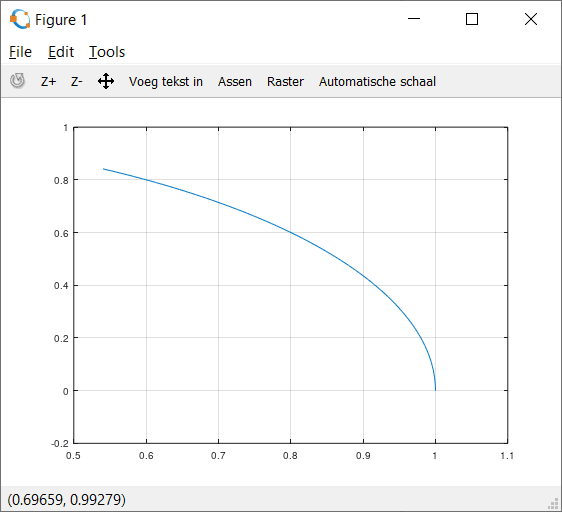So there are a couple of issues:

In your calls to sin, asin, cos and acos you put the ratioX|Y = startPostitionX|Y / orbitRadius. Instead of, as the formula prescribes orbitPeriod*(t - t0)

Also, you make a distiction between t0X and t0Y, which makes no sense to me. There is only one t0, this is a scalar (the time), not a vector.

I'm pretty sure there is no mistake with the ratio. Say for example I have:

0.147i = 0.175 cos (w (t - t0))i. The ratio is simply 0.147/0.175. I do this before computing the inverse cosine so that I can check for floating point inaccuracy and amend if necessary. Without this, sometimes it may return 1.0000001 which would return a NaN math error.

Okay I see your point regarding t0. Let me give you an example of the issue I am experiencing:

Here is an object entering a circular motion. It has an orbital speed of 180°/s and thus a period of 2s. I have set the function to predict the future position at t = 0.5s as soon as it enters the green circle. We can expect it to predict the top of the circle, i.e. 90° of travel (ccw motion).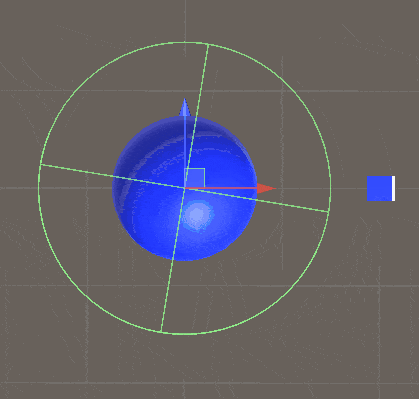Lo and behold, it works as expected using your original formula in #6. (The pink dot is the prediction).

However, if I now enter from a different quadrant, with the exact same parameters, here is what happens: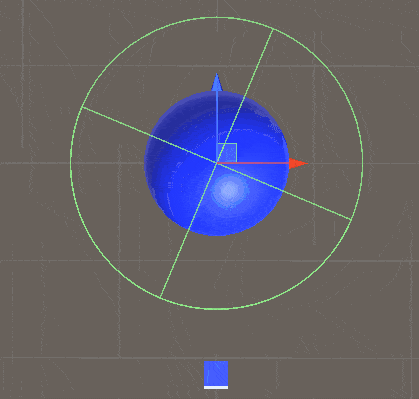It should predict the right hand side of the circle, but you can see it has got the sides wrong. I have tinkered around with this significantly, and the only solution I could find was the tweaking the equations as I outlined in #16 (as reflected in the code block). That appears to always result in correct predictions.

Unless I am computing the original formula wrong, but then surely it wouldn't work correctly in the first case?